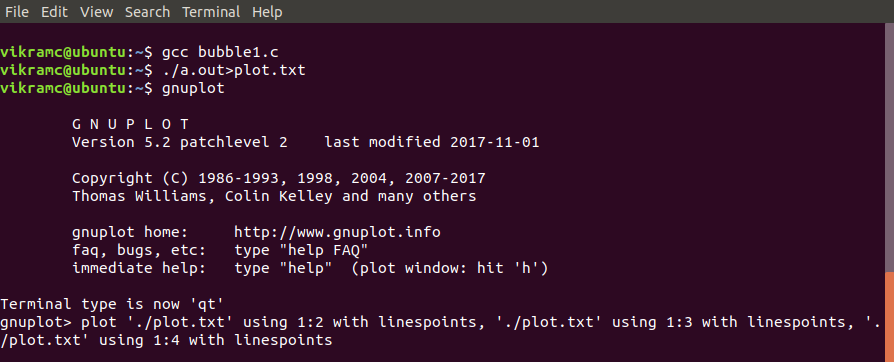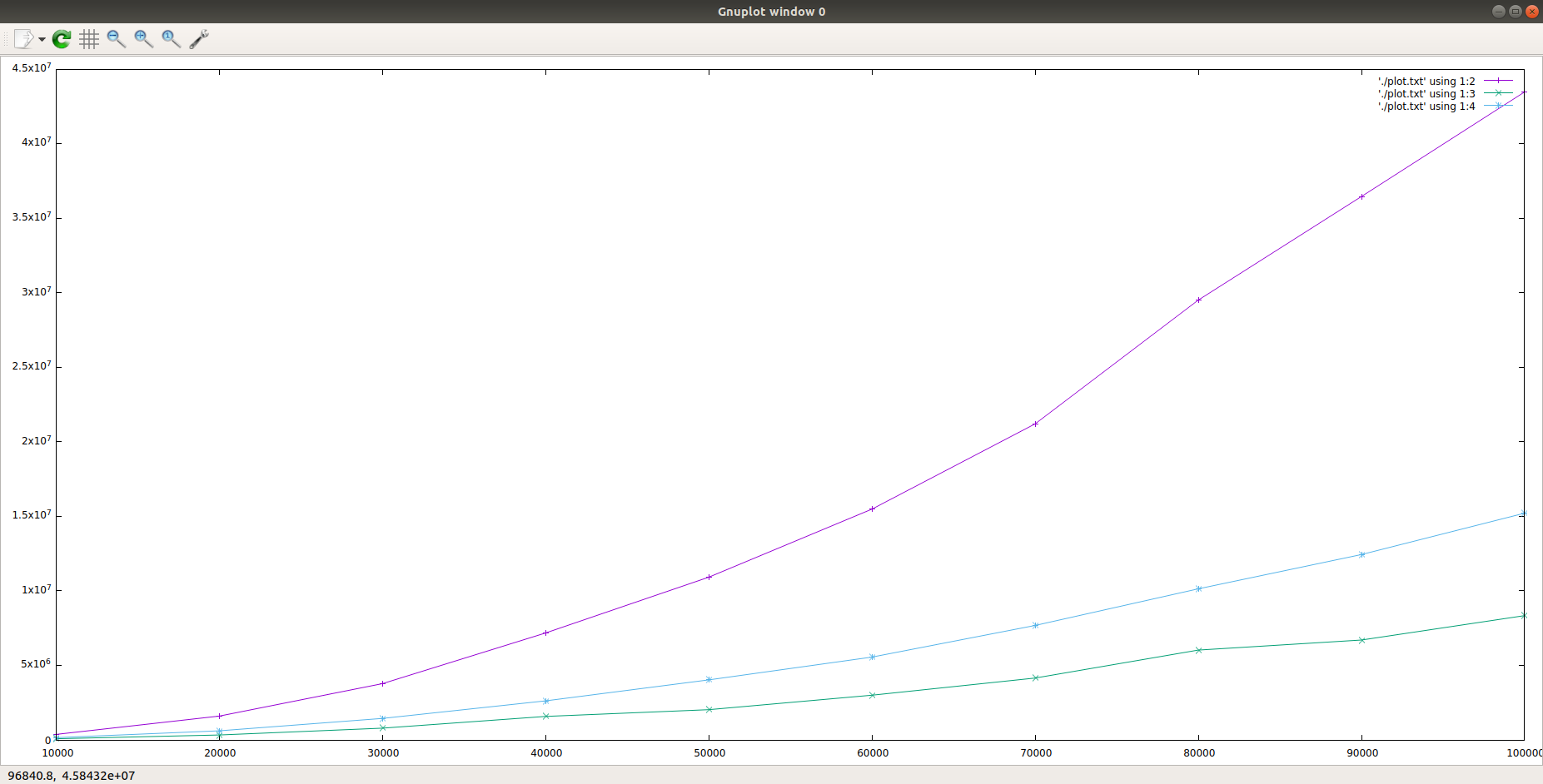# C program for Time Complexity plot of Bubble, Insertion and Selection Sort using Gnuplot

Write a C program to plot and analyze the time complexity of Bubble sort, Insertion sort and Selection sort (using Gnuplot).
As per the problem we have to plot a time complexity graph by just using C. So we will be making sorting algorithms as functions and all the algorithms are given to sort exactly the same array to keep the comparison fair.

Examples:

```Input: randomly generated arrays (using rand() function)
of different sizes as input for sorting.
Output:
A_size, Bubble, Insertion, Selection
10000, 366263, 80736, 152975
20000, 1594932, 332101, 609388
30000, 3773121, 785790, 1441547
40000, 7174455, 1574855, 2620006
50000, 10917061, 2029586, 4025993
60000, 15484338, 2998403, 5556494
70000, 21201561, 4146680, 7678139
80000, 29506758, 6027335, 10131950
90000, 36457272, 6699452, 12436376
100000, 43472313, 8335881, 15208712
```

Approach: We will be using arrays of different sizes to plot the graph between the time taken by the sorting algorithm versus array size. Execution of the program will take some time for sorting arrays of size up to 100000 elements.

Below is the implementation of the above approach:

 `// C program to store time taken by bubble sort, ` `// insertion sort and selection sort ` `// for sorting exactly same arrays. ` ` `  `#include ` `#include ` `#include ` ` `  `// Swap utility ` `void swap(long int* a, long int* b) ` `{ ` `    ``int tmp = *a; ` `    ``*a = *b; ` `    ``*b = tmp; ` `} ` ` `  `// Bubble sort ` `void bubbleSort(long int a[], long int n) ` `{ ` `    ``for (long int i = 0; i < n - 1; i++) { ` `        ``for (long int j = 0; j < n - 1 - i; j++) { ` `            ``if (a[j] > a[j + 1]) { ` `                ``swap(&a[j], &a[j + 1]); ` `            ``} ` `        ``} ` `    ``} ` `} ` ` `  `// Insertion sort ` `void insertionSort(long int arr[], long int n) ` `{ ` `    ``long int i, key, j; ` `    ``for (i = 1; i < n; i++) { ` `        ``key = arr[i]; ` `        ``j = i - 1; ` ` `  `        ``// Move elements of arr[0..i-1], that are ` `        ``// greater than key, to one position ahead ` `        ``// of their current position ` `        ``while (j >= 0 && arr[j] > key) { ` `            ``arr[j + 1] = arr[j]; ` `            ``j = j - 1; ` `        ``} ` `        ``arr[j + 1] = key; ` `    ``} ` `} ` ` `  `// Selection sort ` `void selectionSort(long int arr[], long int n) ` `{ ` `    ``long int i, j, midx; ` ` `  `    ``for (i = 0; i < n - 1; i++) { ` ` `  `        ``// Find the minimum element in unsorted array ` `        ``midx = i; ` ` `  `        ``for (j = i + 1; j < n; j++) ` `            ``if (arr[j] < arr[min_idx]) ` `                ``midx = j; ` ` `  `        ``// Swap the found minimum element ` `        ``// with the first element ` `        ``swap(&arr[midx], &arr[i]); ` `    ``} ` `} ` ` `  `// Driver code ` `int main() ` `{ ` `    ``long int n = 10000; ` `    ``int it = 0; ` ` `  `    ``// Arrays to store time duration ` `    ``// of sorting algorithms ` `    ``double tim1, tim2, tim3; ` ` `  `    ``printf("A_size, Bubble, Insertion, Selection\n"); ` ` `  `    ``// Performs 10 iterations ` `    ``while (it++ < 10) { ` `        ``long int a[n], b[n], c[n]; ` ` `  `        ``// generating n random numbers ` `        ``// storing them in arrays a, b, c ` `        ``for (int i = 0; i < n; i++) { ` `            ``long int no = rand() % n + 1; ` `            ``a[i] = no; ` `            ``b[i] = no; ` `            ``c[i] = no; ` `        ``} ` ` `  `        ``// using clock_t to store time ` `        ``clock_t start, end; ` ` `  `        ``// Bubble sort ` `        ``start = clock(); ` `        ``bubbleSort(a, n); ` `        ``end = clock(); ` ` `  `        ``tim1[it] = ((double)(end - start)); ` ` `  `        ``// Insertion sort ` `        ``start = clock(); ` `        ``insertionSort(b, n); ` `        ``end = clock(); ` ` `  `        ``tim2[it] = ((double)(end - start)); ` ` `  `        ``// Selection sort ` `        ``start = clock(); ` `        ``selectionSort(c, n); ` `        ``end = clock(); ` ` `  `        ``tim3[it] = ((double)(end - start)); ` ` `  `        ``// type conversion to long int ` `        ``// for plotting graph with integer values ` `        ``printf("%li, %li, %li, %li\n", ` `               ``n, ` `               ``(long int)tim1[it], ` `               ``(long int)tim2[it], ` `               ``(long int)tim3[it]); ` ` `  `        ``// increases the size of array by 10000 ` `        ``n += 10000; ` `    ``} ` ` `  `    ``return 0; ` `} `

Output:

```A_size, Bubble, Insertion, Selection
10000, 366263, 80736, 152975
20000, 1594932, 332101, 609388
30000, 3773121, 785790, 1441547
40000, 7174455, 1574855, 2620006
50000, 10917061, 2029586, 4025993
60000, 15484338, 2998403, 5556494
70000, 21201561, 4146680, 7678139
80000, 29506758, 6027335, 10131950
90000, 36457272, 6699452, 12436376
100000, 43472313, 8335881, 15208712
```

Now comes the question that how are we going to plot a graph on x-y coordinates?
For that, we will be using a very simple utility called Gnuplot.
Gnuplot is a portable command-line driven graphing utility for Linux, MS Windows, OSX and many other platforms.

1. First things first how to install Gnuplot.Use this command to install Gnuplot.
`sudo apt-get install gnuplot`
2. After this compile your code and copy the output in a text file using this command.
`./a.out>plot.txt`
3. Open Gnuplot simply using.
`gnuplot`
4. Now the last thing is to plot the graph so use this command to plot the complexity graph.
```plot './plot.txt' using 1:2 with linespoints,
'./plot.txt' using 1:3 with linespoints,
'./plot.txt' using 1:4 with linespoints```

Here is a terminal-snap of commands.Here is the graph of complexity comparing bubble sort(purple curve), insertion sort(green curve) and selection sort(blue curve).Observation:
The average time complexity of all three algorithms is O(n^2) but as the size of input data increases, insertion sort performs far better than bubble sort and slightly better than selection sort.

Attention reader! Don’t stop learning now. Get hold of all the important DSA concepts with the DSA Self Paced Course at a student-friendly price and become industry ready.

My Personal Notes arrow_drop_upCheck out this Author's contributed articles.

If you like GeeksforGeeks and would like to contribute, you can also write an article using contribute.geeksforgeeks.org or mail your article to contribute@geeksforgeeks.org. See your article appearing on the GeeksforGeeks main page and help other Geeks.

Please Improve this article if you find anything incorrect by clicking on the "Improve Article" button below.

Article Tags :
Practice Tags :

1

Please write to us at contribute@geeksforgeeks.org to report any issue with the above content.